# Statistics¶

## Expected Value¶

The predicted value of a variable, calculated as the sum of all possible values (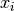) each multiplied by the probability (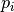) of its occurrence.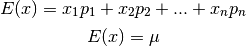The expected value is the mean of a random variable (greek letter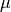).

### Example¶

You pay $2 to roll a six sided dice. If you roll a 6, you get$10. The expected value will tell you how much money you should make in the long run.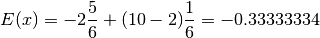So, 5 of the 6 faces is a loosing roll, so you loose $2. You have to pay$2 to play, so your next win is only \$8 if you roll a 6. Therefore you expect to loose 33 cents in the long run if you play this game … house always wins!

## Variance¶

Variance describes how far a set of numbers are spread out from each other from the mean. It is the average of the squared differences from the mean. It is also the covariance of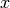with it self.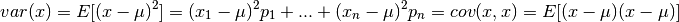Variance is also one of the moments of a distribution.

### Example¶

A perfect die has an expected value and variance of: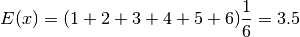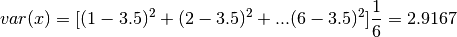Note: The 1/6 above is the probability assuming a fair 6 sided dice.

## Covariance¶

The measure of how two random variables change together.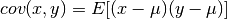For random variables which show similar behavior the sign of the cov is positive. If the random variables act differently, then the sign is negative. The magnitude is hard to interpret unless the cov is normalized, called the correlation coefficient.

## Standard Deviation¶

The standard dev is the square root of the variance.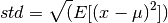### Example¶

The standard dev of the above dice example is:

std(x) = 1.7078


### Example: Octave¶

x = [ 1 2 3 4 5 6 ];
var(x,1) = 2.9167
std(x,1) = 1.7078


Note: The option 1 is passed because the variance and std are taken of the complete set of 6 (N=1). The complete set is also called the Population. The default is that this is a sample of a much larger distribution, so this is just 6 samples. When this is just a sample set, the devisor is now N-1 (i.e., N=5 for this example) and the results are different.

### Example: Python¶

from numpy import *
x = array([1,2,3,4,5,6])
std(x) = 1.7078
var(x) = 2.9167